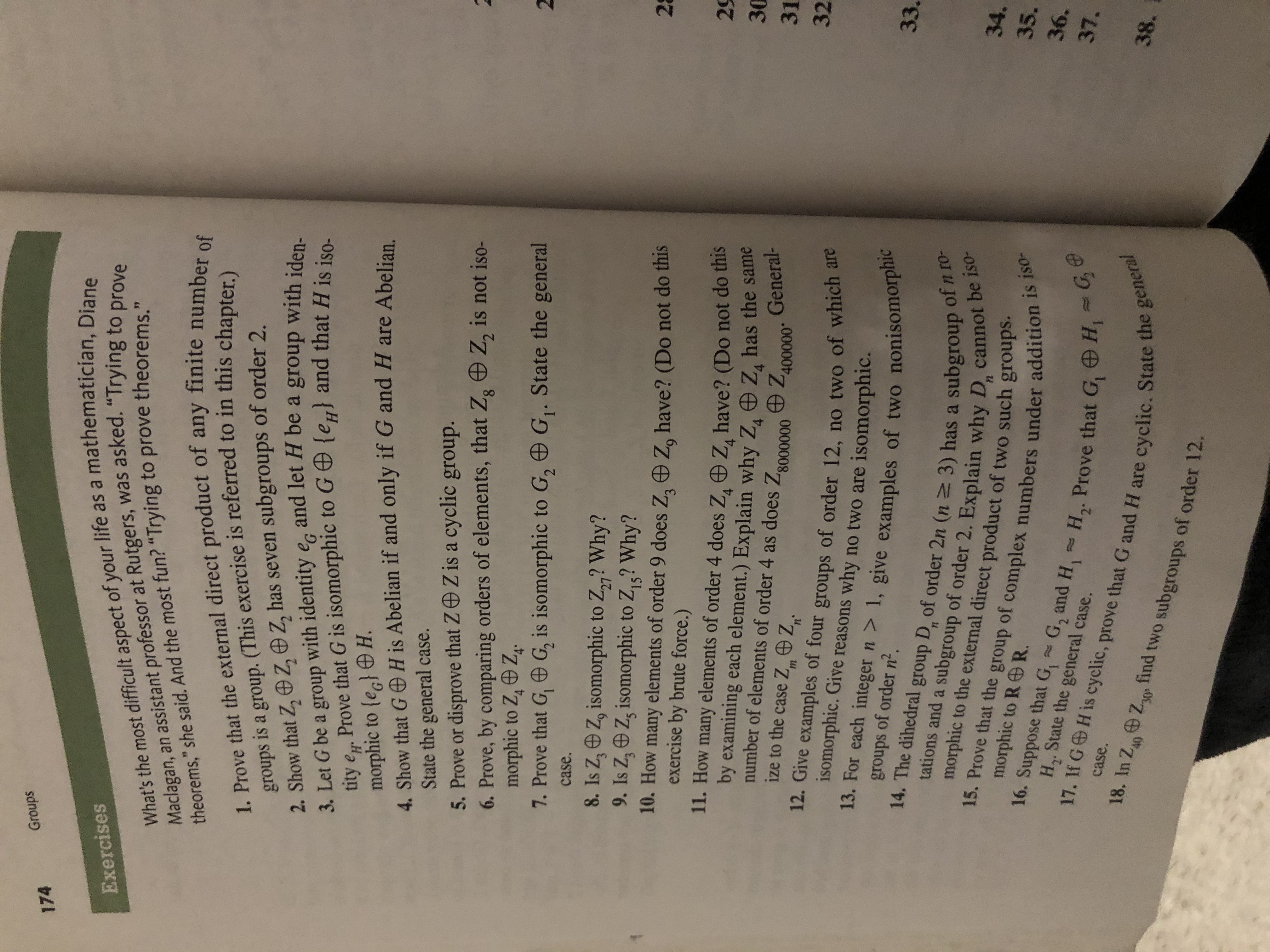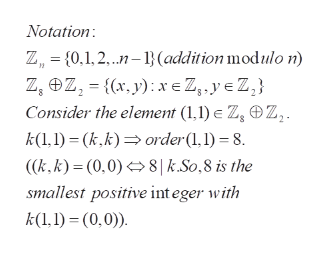# Groups174What's the most difficult aspect of your life as a mathematician, DianeMaclagan, an assistant professor at Rutgers, was asked. "Trying to provetheorems," she said. And the most fun? "Trying to prove theorems."Exercises1. Prove that the external direct product of any finite number ofsa group. (This exercise is referred to in this chapter.)2. Show that Z, Z, Z, has seven subgroups of order 23. Let G be a group with identity e and let H be a group with iden-tity e Prove that G is isomorphic to G{e} and that H is isomorphic to (eg) H4. Show that G H is Abelian if and only if G and H are AbelianState the general case.5. Prove or disprove that Z Z is a cyclic group.6. Prove, by comparing orders of elements, that Zg Z, is not iso-morphic to Z, Z7. Prove that G, G, is isomorphic to G, G,. State the generalgroups284case.8. Is Z,Z, isomorphic to Z2? Why?9. Is Z,Z, isomorphic to Z? Why?10. How many elements of order 9 does Z Z, have? (Do not do this1528exercise by brute force.)11. How many elements of order 4 does Z, Z, have? (Do not do thisby examining each element.) Explain why Z Znumber of elements of order 4 as does Zs00000 Z400000ize to the case Z Z.29has the sameGeneral-30313212. Give examples of four groups of order 12, no two of which areisomorphic. Give reasons why no two are isomorphic.13. For each integer n > 1, give examples of two nonisomorphic14. The dihedral group D, of order 2n (n 3) has a subgroup of n ro-of order n2.groups33.tations and a subgroup of order 2. Explain why D, cannot be iso-15. Prove that the group of complex numbers under addition is iso-16. Suppose that G, G2 and H, H2. Prove that G, H Gmorphic to the external direct product of two such groups.34.morphic to R35.R36.H,. State the general case.17. If GH is cyclic, prove that G and H are37.case.18. In Z438Zcyclic. State the generafind two subgroups of order 12.30

Question

6help_outlineImage TranscriptioncloseGroups 174 What's the most difficult aspect of your life as a mathematician, Diane Maclagan, an assistant professor at Rutgers, was asked. "Trying to prove theorems," she said. And the most fun? "Trying to prove theorems." Exercises 1. Prove that the external direct product of any finite number of sa group. (This exercise is referred to in this chapter.) 2. Show that Z, Z, Z, has seven subgroups of order 2 3. Let G be a group with identity e and let H be a group with iden- tity e Prove that G is isomorphic to G{e} and that H is iso morphic to (eg) H 4. Show that G H is Abelian if and only if G and H are Abelian State the general case. 5. Prove or disprove that Z Z is a cyclic group. 6. Prove, by comparing orders of elements, that Zg Z, is not iso- morphic to Z, Z 7. Prove that G, G, is isomorphic to G, G,. State the general groups 2 8 4 case. 8. Is Z,Z, isomorphic to Z2? Why? 9. Is Z,Z, isomorphic to Z? Why? 10. How many elements of order 9 does Z Z, have? (Do not do this 15 28 exercise by brute force.) 11. How many elements of order 4 does Z, Z, have? (Do not do this by examining each element.) Explain why Z Z number of elements of order 4 as does Zs00000 Z400000 ize to the case Z Z. 29 has the same General- 30 31 32 12. Give examples of four groups of order 12, no two of which are isomorphic. Give reasons why no two are isomorphic. 13. For each integer n > 1, give examples of two nonisomorphic 14. The dihedral group D, of order 2n (n 3) has a subgroup of n ro- of order n2. groups 33. tations and a subgroup of order 2. Explain why D, cannot be iso- 15. Prove that the group of complex numbers under addition is iso- 16. Suppose that G, G2 and H, H2. Prove that G, H G morphic to the external direct product of two such groups. 34. morphic to R 35. R 36. H,. State the general case. 17. If GH is cyclic, prove that G and H are 37. case. 18. In Z4 38 Z cyclic. State the genera find two subgroups of order 12. 30 fullscreen
check_circle

Step 1

To show that there is no isomorphism between the two direct sums , by considering orders of elements in the two groups

Step 2

Observe that there exists an element (in fact (1...help_outlineImage TranscriptioncloseNotation: Z,{0,1,2.n-(addition modulo n) Z, Z,={(x, y) xe 2,yeZ Consider the element (1,1) e Z, OZ,. order (1, 1) 8 k(,1) (k,k) (k,k) = (0,0) 8| k.So,8 is the smallest positive integer with k(l,)(0,0)) fullscreen

### Want to see the full answer?

See Solution

#### Want to see this answer and more?

Solutions are written by subject experts who are available 24/7. Questions are typically answered within 1 hour.*

See Solution
*Response times may vary by subject and question.
Tagged in

### Math